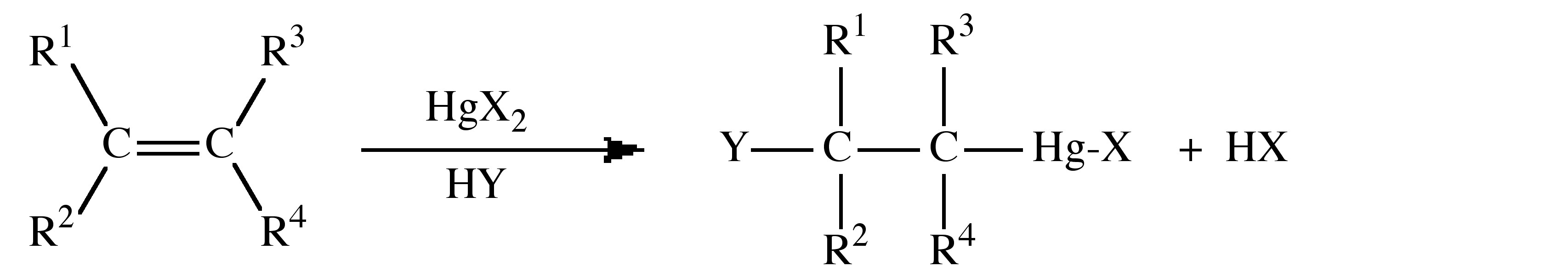# 5.4: Organomercury Compounds

$$\newcommand{\vecs}{\overset { \rightharpoonup} {\mathbf{#1}} }$$ $$\newcommand{\vecd}{\overset{-\!-\!\rightharpoonup}{\vphantom{a}\smash {#1}}}$$$$\newcommand{\id}{\mathrm{id}}$$ $$\newcommand{\Span}{\mathrm{span}}$$ $$\newcommand{\kernel}{\mathrm{null}\,}$$ $$\newcommand{\range}{\mathrm{range}\,}$$ $$\newcommand{\RealPart}{\mathrm{Re}}$$ $$\newcommand{\ImaginaryPart}{\mathrm{Im}}$$ $$\newcommand{\Argument}{\mathrm{Arg}}$$ $$\newcommand{\norm}{\| #1 \|}$$ $$\newcommand{\inner}{\langle #1, #2 \rangle}$$ $$\newcommand{\Span}{\mathrm{span}}$$ $$\newcommand{\id}{\mathrm{id}}$$ $$\newcommand{\Span}{\mathrm{span}}$$ $$\newcommand{\kernel}{\mathrm{null}\,}$$ $$\newcommand{\range}{\mathrm{range}\,}$$ $$\newcommand{\RealPart}{\mathrm{Re}}$$ $$\newcommand{\ImaginaryPart}{\mathrm{Im}}$$ $$\newcommand{\Argument}{\mathrm{Arg}}$$ $$\newcommand{\norm}{\| #1 \|}$$ $$\newcommand{\inner}{\langle #1, #2 \rangle}$$ $$\newcommand{\Span}{\mathrm{span}}$$$$\newcommand{\AA}{\unicode[.8,0]{x212B}}$$

## Synthesis

The most common routes to organomercury compounds involve the direct reaction of mercury with an alkyl iodide (Equation 5.4.1) to form the mercury analog of a Grignard reagent.

$\text{Hg + RX} \rightarrow \text{RHgX}$

The subsequent reaction of RHgI with potassium cyanide yields the appropriate dialkyl mercury derivative (Equation 5.4.2).

$\text{2 RHgI + 2 KCN} \rightarrow \text{R}_2\text{Hg + Hg + 2 KI + (NC)}_2$

### Solvomercuration

The general process of solvomercuration involves the addition of HgX2 across an alkene double (or alkyne triple) bond in the presence of a solvent. Solvomercuration applies where HY, from Equation 5.4.3, are part of the solvent system, e.g., in water the process can be described as hydroxymercuration.The addition to the alkene occurs with Markovnikoff addition, i.e., through the formation of the most stable carbonium ion resulting with the mercury adding to the less substituted carbon. The Hg-C bond can be cleaved by the addition of NaBH4 to yield the C-H bond. It is common to employ mercury acetate, Hg(OAc)2 (OAc = O2CCH3), with subsequent reaction with NaCl or NaI to form the halide, rather than the mercury halide directly. However, the acetate can also act as a neucleophile resulting in a mixture of products. The order of reactivity for alkenes follows the trend:

$\text{R}_2\text{C=CH}_2\text{ > R(H)C=CH}_2 \text{ >} cis \text{ R(H)C=C(H)R > } trans \text{ R(H)C=C(H)R > R}_2\text{C=C(H)R > R}_2\text{C=CR}_2$

This is due to a combination of steric and electronic effects. The addition occurs in a trans/anti fashion.Mercury(II) halides dissociate in polar solvents (Equation 5.4.4) and this species is commonly associated with the initial reaction step.

$\text{HgX}_2 \leftrightharpoons \text{HgX}^+ \text{ + X}^-$

The mechanism of solvomercuration is best described by the reaction shown in.

Evidence for the mechanism is twofold:

1. The addition is exclusively trans.
2. No rearrangement is observed even for tBu(H)C=C(H)tBu whose carbonium ion is known to undergo rearrangement.

Isotope studies indicate that the C-O bond formation is present in the transition state. The mercury bridge in the transition state may not be symmetrical, but the structure is similar to the addition of Br+ and AuX+ to alkenes.Proposed transition state for the solvomercuration reaction where the solvent is methanol.

The actual reaction was originally carried out with Hg(OAc)2 in benzene at 110 °C for several hours in acetic acid solution. It was found that the reaction was catalyzed by the presence of HClO4, H2SO4, and HNO3, which were found to replace the acetate ion. The reaction rate is also increased by 690,000 times by the use of Hg(O2CCF3)2 in HO2CCF3.

The solvomercuration of alkynes gives alkenylmercury compounds, but the reaction is more sluggish than for the reaction with alkenes, and the product is always the trans isomer.

### Mercuration of aromatic compounds

Mercuration is an electrophilic aromatic substitution reaction that is possible for most 2n+2 π-electron species. Evidence for the π-complex intermediate is indicated by UV spectroscopy, which shows an increase in the region 280 – 320 nm for the reaction of aromatic compounds with Hg(O2CCF3)2 in HO2CCF3. The σ-complex has detected in liquid SO2.As a preparative method mercuration suffers from alack of selectivity, including an isomerization from para substitution at low temperature to meta substitution at high temperatures.

## Structure

Dialkyl mercury compounds, R2Hg, are generally air stable and show little Lewis acid behavior. They are monomeric colorless liquids or low melting solids, e.g., Bp = 92.5 °C for Me2Hg. No solubility is observed in water, except for (F3C)2Hg (Equation 5.4.5).

$\text{(F}_3\text{C)}_2\text{Hg + H}_2\text{O} \leftrightharpoons \text{(F}_3\text{C)}_2\text{Hg(OH)}^- \text{ + H}^+$

The hybridization at mercury involves the 6s and 6p orbitals; however, the 5d may be involved. X-ray crystal structures for both R2Hg and RHgX show linear structures. In general R2Hg compounds are very weak Lewis acids, but adduct are formed if the alkyl group is sufficiently electron withdrawing, e.g., (C6F5)2Hg. The geometry of the Lewis acid-base complex is not triangular as predicted from VSEPR theory, but a T-shape. The distortion from a linear C-Hg-C unit is minor. For example, in (C6F5)2Hg the C-Hg-C angle is 176.2°, while in [(C6F5)2Hg]2(diars), where diars = Ph2AsCH2CH2AsPh2, the same angle is 173°. Furthermore, the donor atom-Hg bond distance (e.g., As-Hg = 3.4 Å) is only slightly shorter than the sum of the van der Waal radii (e.g., 3.5 Å).